# 二次算术程序：关于零知识证明的论述

1- 你知道zk-SNARKs和他的大致原理；

2- 你有足够的数学知识，能理解一些基本的多项式知识。(如 if P(x)+ Q(x)=(P + Q)(x)，P和Q代表多项式，如果你对这类多项式表述方式已经非常熟悉，说明你符合继续阅读的要求)。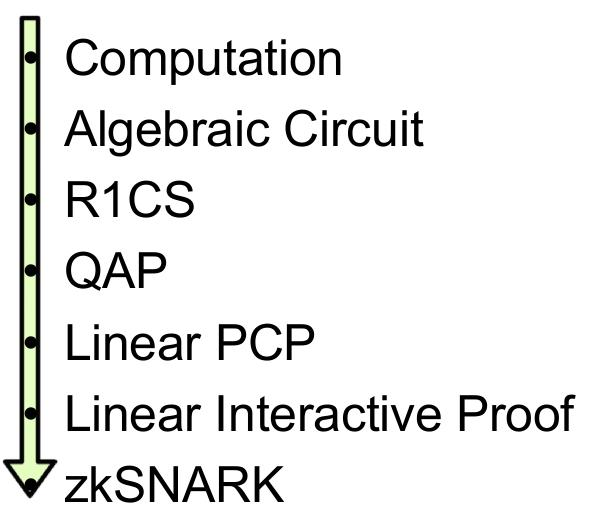zk-SNARK知识管道图，Eran Tromer绘制

def qeval(x):
y = x**3
return x + y + 5

### 第一步：压扁

1- x = y （y可以是变量或数字)
2- x = y(op)z (op可以+,-,*,/，y和z可以是变量，数字或子表达式)。

sym_1 = x * x
y = sym_1 * x //相当于实现了幂函数y = x**3
sym_2 = y + x
~out = sym_2 + 5

### 第二步：转为R1CS

s . a * s . b - s . c = 0

a = (5,0,0,0,0,1)，
b = (1,0,0,0,0,0)，
c = (0,0,1,0,0,0)，
s = (1,3,35,9,27,30)，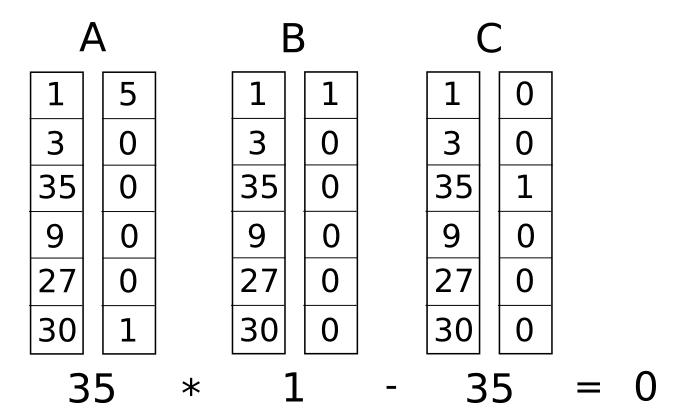（译者注：第一个35=1*5 + 30*1，第二个35=35 * 1）

'~one', 'x', '~out', 'sym_1', 'y', 'sym_2'

sym_1 = x * x，即 x*x - sym_1 = 0

a = [0, 1, 0, 0, 0, 0]
b = [0, 1, 0, 0, 0, 0]
c = [0, 0, 0, 1, 0, 0]

y = sym_1 * x，即 sym_1 * x - y = 0

a = [0, 0, 0, 1, 0, 0]
b = [0, 1, 0, 0, 0, 0]
c = [0, 0, 0, 0, 1, 0]

sym_2 = y + x，加法门需要转换为：(x + y) * 1 - sym_2 = 0

a = [0, 1, 0, 0, 1, 0]
b = [1, 0, 0, 0, 0, 0] 对应常量1，用~one位
c = [0, 0, 0, 0, 0, 1]

~out = sym_2 + 5，即 (sym_2 + 5) * 1 - ~out = 0

a = [5, 0, 0, 0, 0, 1]
b = [1, 0, 0, 0, 0, 0]
c = [0, 0, 1, 0, 0, 0]

s = [1, 3, 35, 9, 27, 30]

A
[0, 1, 0, 0, 0, 0]
[0, 0, 0, 1, 0, 0]
[0, 1, 0, 0, 1, 0]
[5, 0, 0, 0, 0, 1]

B
[0, 1, 0, 0, 0, 0]
[0, 1, 0, 0, 0, 0]
[1, 0, 0, 0, 0, 0]
[1, 0, 0, 0, 0, 0]

C
[0, 0, 0, 1, 0, 0]
[0, 0, 0, 0, 1, 0]
[0, 0, 0, 0, 0, 1]
[0, 0, 1, 0, 0, 0]

### 第三步：从R1CS 到 QAP

y = (x - 2) * (x - 3)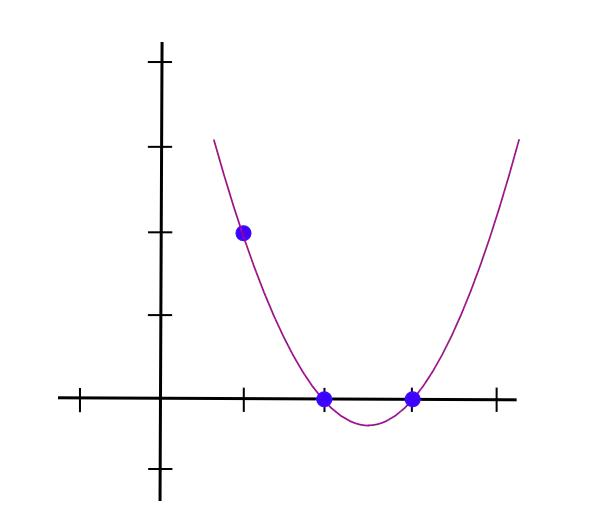y = (x - 2) * (x - 3) * 3 / ((1 - 2) * (1 - 3))

y = 1.5 * x**2 - 7.5 * x + 9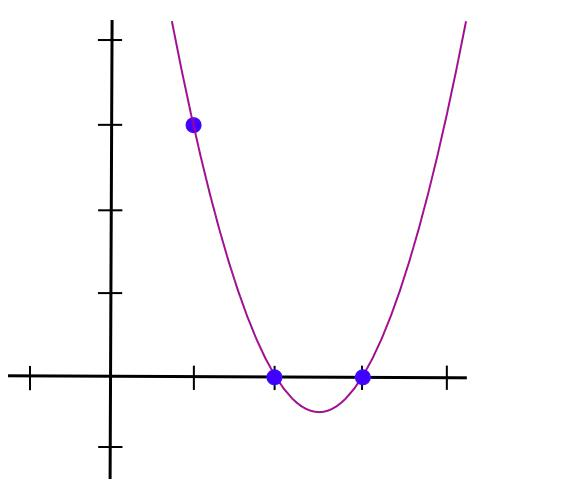y = 1.5 * x**2 - 5.5 * x + 7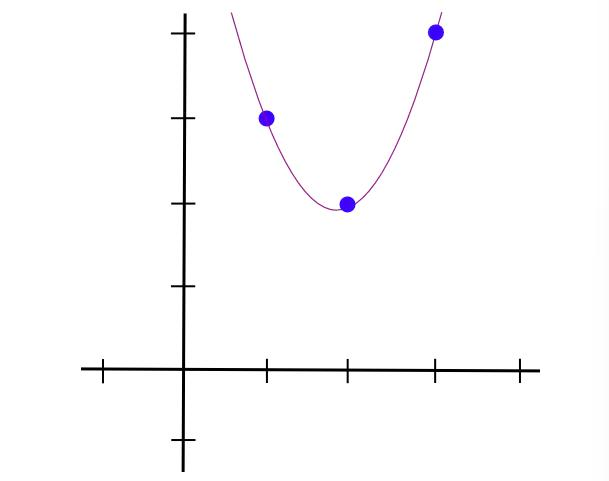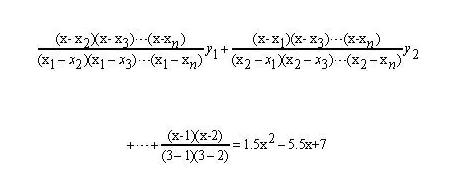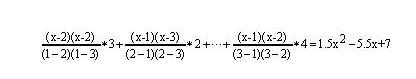A polynomials
[-5.0, 9.166, -5.0, 0.833]
[8.0, -11.333, 5.0, -0.666]
[0.0, 0.0, 0.0, 0.0]
[-6.0, 9.5, -4.0, 0.5]
[4.0, -7.0, 3.5, -0.5]
[-1.0, 1.833, -1.0, 0.166]

B polynomials
[3.0, -5.166, 2.5, -0.333]
[-2.0, 5.166, -2.5, 0.333]
[0.0, 0.0, 0.0, 0.0]
[0.0, 0.0, 0.0, 0.0]
[0.0, 0.0, 0.0, 0.0]
[0.0, 0.0, 0.0, 0.0]

C polynomials
[0.0, 0.0, 0.0, 0.0]
[0.0, 0.0, 0.0, 0.0]
[-1.0, 1.833, -1.0, 0.166]
[4.0, -4.333, 1.5, -0.166]
[-6.0, 9.5, -4.0, 0.5]
[4.0, -7.0, 3.5, -0.5]

(0, 1, 0, 0, 0, 0),
(0, 1, 0, 0, 0, 0),
(0, 0, 0, 1, 0, 0),
...

### 第四步：检查QAP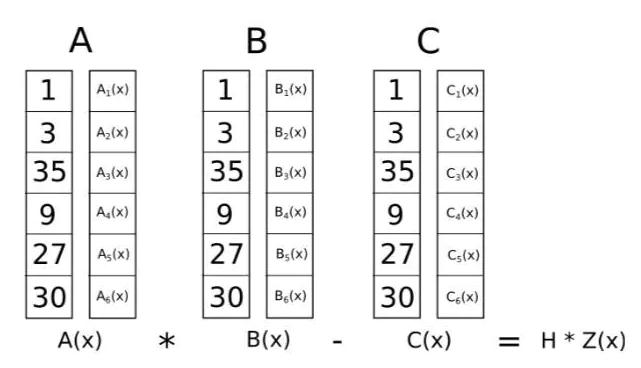Z定义为(x - 1) * (x - 2) * (x - 3)…-最简单的多项式，在所有对应逻辑门的点上都等于0。这是代数的一个基本事实任何多项式在所有这些点上等于零都必须是这个最小多项式的倍数，如果一个多项式是Z的倍数那么它在任何这些点上的值都是零；这种对等使我们的工作容易得多。

A . s = [43.0, -73.333, 38.5, -5.166]
B . s = [-3.0, 10.333, -5.0, 0.666]
C . s = [-41.0, 71.666, -24.5, 2.833]
（译者注：以上计算过程：
43.0 = -5 * 1 + 8 * 3 + 0 * 35 - 6 * 9 + 4 * 27 - 1 * 30，
-73.333 = 9.166 * 1 - 11.333 * 3 + 0 * 35 + 9.5 * 9 - 7 * 27 + 1.833 * 30,
...
-3 = 3 * 1 - 2 * 3 + 0 * 35 + 0 * 9 + 0 * 27 + 0 * 30
...)

t = [-88.0, 592.666, -1063.777, 805.833, -294.777, 51.5, -3.444]
（译者注：计算过程：
A . s = [43.0, -73.333, 38.5, -5.166] = -5.166 * x3 + 38.5 * x2 - 73.333 * x + 43,
B . s = [-3.0, 10.333, -5.0, 0.666] = 0.666 * x3 - 5 * x2 + 10.333 * x - 3.0,
C . s = [-41.0, 71.666, -24.5, 2.833] = 2.833 * x3 - 24.5 * x2 + 71.666 * x - 41.0
A . s * B . s - C . s 就是上面多项式的计算，计算后，按幂从低到高排列系数，得到： [-88.0, 592.666, -1063.777, 805.833, -294.777, 51.5, -3.444]

Z = (x - 1) * (x - 2) * (x - 3) * (x - 4)

Z = [24, -50, 35, -10, 1]

h = t / Z = [-3.666, 17.055, -3.444]

h必须是没有任何余数的整除。ChainCatcher 与创新者共建Web3世界